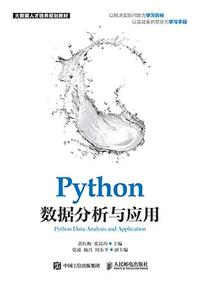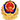(17 封私信 / 84 条消息) Python 中既然可以直接通过父类名调用父类方法为什么还会存在 super 函数？ - 知乎

84

17# # Python 中既然可以直接通过父类名调用父类方法为什么还会存在 super 函数？

[

Python

](//www.zhihu.com/topic/19552832)

# # Python 中既然可以直接通过父类名调用父类方法为什么还会存在 super 函数？

``````class Child(Parent):
def __init__(self):
Parent.__init__(self)
``````

314

38,851

​邀请回答

​好问题 2

​1 条评论

​分享

#### # 13 个回答laike9m

451 人赞同了该回答

``````def super(cls, inst):
mro = inst.__class__.mro()
return mro[mro.index(cls) + 1]
``````

1. inst 负责生成 MRO 的 list
2. 通过 cls 定位当前 MRO 中的 index, 并返回 mro[index + 1]

MRO 全称 Method Resolution Order，它代表了类继承的顺序。后面详细说。

``````class Root(object):
def __init__(self):
print("this is Root")

class B(Root):
def __init__(self):
print("enter B")
# print(self)  # this will print <__main__.D object at 0x...>
super(B, self).__init__()
print("leave B")

class C(Root):
def __init__(self):
print("enter C")
super(C, self).__init__()
print("leave C")

class D(B, C):
pass

d = D()
print(d.__class__.__mro__)
``````

``````enter B
enter C
this is Root
leave C
leave B
(<class '__main__.D'>, <class '__main__.B'>, <class '__main__.C'>, <class '__main__.Root'>, <type 'object'>)
``````

``````super(B, self).__init__()
``````

``````(<class '__main__.D'>, <class '__main__.B'>, <class '__main__.C'>, <class '__main__.Root'>, <type 'object'>)
``````

--
** 更新，回答

@裘堪将

``````class A(object) :
def __init__(self, strName, *args) :
self.name = strName
super(A, self).__init__(*args)

class B(object) :
def __init__(self, iID) :
self.ID= iID

class C(A, B):
def __init__(self, *args)
super(C, self).__init__(*args)
``````

​赞同 451​​106 条评论

​分享

​收藏​喜欢Chromecaimaoy

15 人赞同了该回答

``````class Base(object):
def __init__(self):
print('Base.__init__')

class A(Base):
def __init__(self):
print('A.__init__ begin')
Base.__init__(self)
print('A.__init__ end')

class B(Base):
def __init__(self):
print('B.__init__ begin')
Base.__init__(self)
print('B.__init__ end')

class C(A,B):
def __init__(self):
print('C.__init__ begin')
A.__init__(self)
B.__init__(self)
print('C.__init__ end')

c = C()
print C.__mro__
``````

``````C.__init__ begin
A.__init__ begin
Base.__init__
A.__init__ end
B.__init__ begin
Base.__init__
B.__init__ end
C.__init__ end
(<class '__main__.C'>, <class '__main__.A'>, <class '__main__.B'>, <class '__main__.Base'>, <type 'object'>)
``````

``````class Base(object):
def __init__(self):
print('Base.__init__')

class A(Base):
def __init__(self):
print('A.__init__ begin')
super(A, self).__init__()
print('A.__init__ end')

class B(Base):
def __init__(self):
print('B.__init__ begin')
super(B, self).__init__()
print('B.__init__ end')

class C(A,B):
def __init__(self):
print('C.__init__ begin')
super(C, self).__init__()  # Only one call to super() here
print('C.__init__ end')

c = C()
print C.__mro__
``````

``````C.__init__ begin
A.__init__ begin
B.__init__ begin
Base.__init__
B.__init__ end
A.__init__ end
C.__init__ end
(<class '__main__.C'>, <class '__main__.A'>, <class '__main__.B'>, <class '__main__.Base'>, <type 'object'>)
``````

​赞同 15​​2 条评论

​分享

​收藏​喜欢Chrome36 人赞同了该回答

super 是用来解决多重继承问题的，直接用类名调用父类方法在使用单继承的时候没问题，但是如果使用多继承，会涉及到查找顺序（MRO）、重复调用（钻石继承）等种种问题。

• 普通类多重继承只能有一个普通父类和若干个 Mixin 类（保持主干单一）
• Mixin 类不能继承普通类（避免钻石继承）
• Mixin 类应该单一职责（参考 Java 的 interface 设计，Mixin 和此极其相似，只不过附带实现而已）

​赞同 36​​10 条评论

​分享

​收藏​喜欢Chromeest

​赞同​​添加评论

​分享

​收藏​喜欢Chromeahuigo

3 人赞同了该回答

1. python 实现了多继承, 这个继承的顺序叫 MRO(可以看看 print(class.__mro__))
2. super 只是提供了一种调用父类方法. 它并不是实现多继承的!
super 调用父类时, 其实就是搜索: class.__mro__

​赞同 3​​2 条评论

​分享

​收藏​喜欢Chrome2 人赞同了该回答

http://blog.csdn.net/qq_14898613/article/details/53792270

​赞同 2​​添加评论

​分享

​收藏​喜欢Chrome``````def super(cls, inst):
mro = inst.__class__.mro()
return mro[mro.index(cls) + 1]
``````

​赞同​​添加评论

​分享

​收藏​喜欢Chrome__mro__及菱形继承问题

​赞同​​添加评论

​分享

​收藏​喜欢Chrome2 人赞同了该回答

http://blog.csdn.net/johnsonguo/article/details/585193

​赞同 2​​2 条评论

​分享

​收藏​喜欢Chrome@laike9m

​赞同​​2 条评论

​分享

​收藏​喜欢Chromeinheritance - Understanding Python super() with __init__() methods

​赞同​​添加评论

​分享

​收藏​喜欢Chromeruiming tang

1 人赞同了该回答

​赞同 1​​添加评论

​分享

​收藏​喜欢ChromeRogue like 游戏、 旅游 爱好者

2 人赞同了该回答

super(A,self).func 不是把 A 的父类的 func 执行，而是把 A 的父类的类类型序列中的所有类型的 func 都执行一次。

​赞同 2​​7 条评论

​分享

​收藏​喜欢Chrome

​写回答

python 应该怎么记各种函数名？ 7 个回答

[Idle Ideas in 1905

Jerome Klapka Jerome

0 人读过​阅读

](/pub/book/120125079)[Python 地理空间分析指南（第 2 版）

110 人读过​阅读

](/pub/book/119583521)[Python 数据分析与应用

113 人读过​阅读

](/pub/book/119582426)京公网安备 11010802020088 号

（京）- 非经营性 - 2017 - 0067 违法和不良信息举报：010-82716601